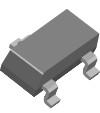< id="6e2kg">< id="6e2kg">< id="6e2kg">< id="6e2kg">< id="6e2kg">< id="6e2kg">< id="6e2kg">< id="6e2kg">< id="6e2kg">< id="6e2kg">< id="6e2kg">< id="6e2kg">< id="6e2kg">< id="6e2kg">< id="6e2kg">< id="6e2kg">< id="6e2kg">< id="6e2kg">< id="6e2kg">< id="6e2kg">< id="6e2kg">< id="6e2kg">< id="6e2kg">< id="6e2kg">< id="6e2kg">< id="6e2kg">< id="6e2kg">< id="6e2kg">< id="6e2kg">< id="6e2kg">< id="6e2kg">< id="6e2kg">< id="6e2kg">< id="6e2kg">< id="6e2kg">< id="6e2kg">< id="6e2kg">< id="6e2kg">< id="6e2kg">< id="6e2kg">< id="6e2kg">< id="6e2kg">< id="6e2kg">< id="6e2kg">< id="6e2kg">< id="6e2kg">< id="6e2kg">< id="6e2kg">< id="6e2kg">< id="6e2kg"> < id="6e2kg">< id="6e2kg">< id="6e2kg">< id="6e2kg">< id="6e2kg">< id="6e2kg">< id="6e2kg">< id="6e2kg">< id="6e2kg">< id="6e2kg">< id="6e2kg">< id="6e2kg">< id="6e2kg">< id="6e2kg">< id="6e2kg">< id="6e2kg">< id="6e2kg">< id="6e2kg">< id="6e2kg">< id="6e2kg">< id="6e2kg">< id="6e2kg">< id="6e2kg">< id="6e2kg">< id="6e2kg">< id="6e2kg">< id="6e2kg">< id="6e2kg">< id="6e2kg">< id="6e2kg">< id="6e2kg">< id="6e2kg">< id="6e2kg">< id="6e2kg">< id="6e2kg">< id="6e2kg">< id="6e2kg">< id="6e2kg">< id="6e2kg">< id="6e2kg">< id="6e2kg">< id="6e2kg">< id="6e2kg">< id="6e2kg">< id="6e2kg">< id="6e2kg">< id="6e2kg">< id="6e2kg">< id="6e2kg">< id="6e2kg"> < id="6e2kg">< id="6e2kg">< id="6e2kg">< id="6e2kg">< id="6e2kg">< id="6e2kg">< id="6e2kg">< id="6e2kg">< id="6e2kg">< id="6e2kg">< id="6e2kg">< id="6e2kg">< id="6e2kg">< id="6e2kg">< id="6e2kg">< id="6e2kg">< id="6e2kg">< id="6e2kg">< id="6e2kg">< id="6e2kg">< id="6e2kg">< id="6e2kg">< id="6e2kg">< id="6e2kg">< id="6e2kg">< id="6e2kg">< id="6e2kg">< id="6e2kg">< id="6e2kg">< id="6e2kg">< id="6e2kg">< id="6e2kg">< id="6e2kg">< id="6e2kg">< id="6e2kg">< id="6e2kg">< id="6e2kg">< id="6e2kg">< id="6e2kg">< id="6e2kg">< id="6e2kg">< id="6e2kg">< id="6e2kg">< id="6e2kg">< id="6e2kg">< id="6e2kg">< id="6e2kg">< id="6e2kg">< id="6e2kg">< id="6e2kg">型号： MMBT3904 厂商：Fairchild Semiconductor 标准：分类： 半导体 ， 分离式半导体 描述： transistors bipolar - bjt sot-23 npn gen pur PDF： 预览 报错 收藏 赞

Datasheet下载地址

 本地下载 >> 厂商下载 >> 厂商下载2 >>

MMBT3904的详细信息

Manufacturer： Fairchild Semiconductor Transistors Bipolar - BJT Yes Single NPN 60 V 40 V 6 V 0.3 V 0.2 A 300 MHz + 150 C SMD/SMT SOT-23 Fairchild Semiconductor 0.2 A 100 300 625 mW - 55 C Reel MMBT3904 3000 MMBT3904_NL 60 mg
October 2011
2N3904 / MMBT3904 / PZT3904
NPN General Purpose Amplifier
Features
? This device is designed as a general purpose amplifier and switch.
? The useful dynamic range extends to 100 mA as a switch and to 100 MHz as an amplifier.
PZT3904
2N3904
MMBT3904
C
C
E
E
C
B
TO-92
SOT-23
B
SOT-223
Mark:1A
EBC
Absolute Maximum Ratings* T = 25°C unless otherwise noted
a
Symbol
Parameter
Collector-Emitter Voltage
Value
Units
V
V
V
V
40
60
CEO
CBO
EBO
Collector-Base Voltage
V
Emitter-Base Voltage
6.0
V
I
Collector Current - Continuous
Operating and Storage Junction Temperature Range
200
mA
°C
C
T T
-55 to +150
J, stg
* These ratings are limiting values above which the serviceability of any semiconductor device may be impaired.
NOTES:
1) These ratings are based on a maximum junction temperature of 150 degrees C.
2) These are steady state limits. The factory should be consulted on applications involving pulsed or low duty cycle
operations.
Thermal Characteristics T = 25°C unless otherwise noted
a
Max.
Symbol
Parameter
Units
2N3904
*MMBT3904
**PZT3904
Total Device Dissipation
625
5.0
350
2.8
1,000
8.0
mW
mW/°C
P
D
Derate above 25°C
R
R
Thermal Resistance, Junction to Case
Thermal Resistance, Junction to Ambient
83.3
200
°C/W
°C/W
θJC
357
125
θJA
* Device mounted on FR-4 PCB 1.6" X 1.6" X 0.06".
** Device mounted on FR-4 PCB 36 mm X 18 mm X 1.5 mm; mounting pad for the collector lead min. 6 cm .
2
? 2011 Fairchild Semiconductor Corporation
2N3904 / MMBT3904 / PZT3904 Rev. B0
www.fairchildsemi.com
1